International Journal of Geomagnetism and Aeronomy
Vol. 3, No. 3, February 2003

# Relation of cyclic variations of the solar wind and interplanetary magnetic field to the cycles of solar magnetic fields

Yu. R. Rivin and L. I. Gromova

Institute of Terrestrial Magnetism, Ionosphere, and Radio Wave Propagation, Troitsk, Russia

### Abstract

Annual mean values of dynamical parameters of the solar wind and interplanetary magnetic field during 1964-1996 and the errors of their determination were obtained from the data of the King catalogue electron version presented on the Internet. The 11-year cycles of all characteristics of the interplanetary plasma and their relation to cycles of various solar magnetic fields were analyzed from them. Using this analysis, the difference in cycles of various characteristics of the interplanetary medium is demonstrated. Attention is paid to systematic discrepancies in cycling variations of the plasma density before and after the beginning of the 1970s and to nonadequacy of variations of the initial interplanetary magnetic field vector to variations of its components.

### 1. Introduction

The current knowledge on plasma flows from the Sun (these flows will below be called solar wind, the definition being broader than the Parker  definition which referred to the plasma flow related only to the corona hydrodynamical expansion into the interplanetary space: quiet solar wind with a velocity of300 km s -1 ) shows that the flows are rather complicated formations which are influenced by the variations of the large-scale solar magnetic fields including the cyclic variation with T11 years. The variation of the annual dynamical parameters of the solar wind (SW) and also "frozen" into it interplanetary magnetic field (IMF, BI ) are below analyzed from these positions. The relation (different for different parameters) between the cyclic variations of the solar magnetic fields and interplanetary medium parameters is demonstrated, and some features of the experimental data in the King catalogue which complicate their analysis are indicated.

### 2. Dynamical Parameters of the PlasmaFigure 1
The initial and smoothed annual values of three dynamical parameters of solar wind (the velocity V, density n, and momentum nV ) calculated for 1964-1996 from the data of the King catalogue (http://nssdc.gsfc.nasa.gov/omniweb) and Rivin  are shown in Figure 1a. The annual parameters and their root mean square error ( s ) were determined in the calculation, the smoothing being performed with a running interval ( L = 3 years). Figure 1a shows the errors only for the initial curve of the solar wind velocity. Their average value for the entire temporal interval iss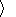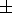10 km s-1; this value is almost by a factor of 1.5 higher in 1986-1996 than earlier during 1964-1985. The values of s for the plasma density has smaller representation scale (s0.5 cm -3 and slightly changes in the entire temporal interval).Figure 2
The comparison of the momentum variations with the velocity and density variations shown in Figure 1a and also the analyzes of the dependencies nV(t) = f(V), nV(t) = f(n) shown in Figure 2 make it possible to conclude that the cyclic variations of nV are not an independent characteristic since they are determined mainly by the cyclic variations of the plasma density: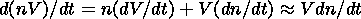(1)

where Vconst, and in the following consideration the main attention should be paid only to analyzes of V and  n.Figure 3
Figure 3 shows the spectra of three dynamical parameters of the plasma. They were obtained by an expansion of the curves in Figure 1a into Fourier series with fractional harmonics. The harmonic with T10-11 years is quite visually (in comparison with other harmonics) seen in the spectrum of V , the spectra of n and nV are distorted by the lower-frequency (than the cycle) variation which may be related to the variations of these two parameters before the beginning of the 1970s (Figure 1a). Its exclusion (by shortening of the series expanded into spectrum) shows presence of a harmonic with T11 years (which is visually seen in the initial data (Figure 1a)) in the variations of these two parameters. The nature of the trend in curve n is not probably related to cyclisity, that fact being discussed below.

Figure 1b shows (smoothed by the same   L ) curves of the annual values of: (1) the Wolf numbers ( W ) as the characteristic of the cyclic variation of the toroidal component of the solar magnetic field 1B generated above the basic of the convective zone, and (2) the magnitude of the radial component of the large-scale magnetic field BR, 2B generated within the basis of the convective zone, and the field of the Sun as a star |Bs| which plays a determining role in processes in the interplanetary medium. Obridko and Rivin  and Rivin [1998a, 1998b, 1999a, 1999b] discussed these characteristics in detail and called the former and latter fields dipole and quadrupole, respectively. A complete comparison of the cycles of these magnetic fields with the interplanetary medium parameters is difficult because of the short series of observations (2-3 cycles). However to distinguish cycles of various fields, the difference in their amplitude modulation was taken into account: for BR the amplitude of cycle 22 is several times lower than the amplitude of cycle 21, for W and Bs the amplitudes in both cycles are approximately equal, or the amplitude of Bs is even slightly higher in cycle 22. These differences and also the phase variations are used in the interpretation below.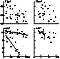Figure 4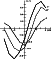Figure 5
The comparison of cyclic variations of V and n with the cycles of the large-scale solar magnetic fields was carried out on the correlation plane (Figure 4) and by calculation of inter-correlation function r(t) (Figure 5). Taking into account the spectra in Figure 3, one can derive the following conclusions from this comparison:

1. In the main dynamical parameters of SW there exists a ~11-year cycle which is mainly in antiphase to the cyclic variations of BR . Its amplitude decreases in n and V from cycle 20 to cycle 22 and the spectral amplitude exceeds by several times the amplitudes of other harmonics including the harmonics with T22 years.

2. The cycle with T11 years in the data on V is additionally shifted in phase by 2-3 years (it lags) relative the cycle of BR , that is, by ~3-4 years relative to the W cycle. Its spectral amplitude for 3 cycles is ~20 km s-1 and that is only slightly higher thans.

3. The properties of the ~11-year cycle in n till the beginning of the 1970s are considerably different from the properties of the following two cycles (on the n-W plane in Figure 3 the data for the years before the beginning of the 1970s form such a point distribution which is approximated by a straight line having essentially different coefficients than those for the following cycles). That requires individual consideration of this parameter before and after the years indicated. It is possible that this difference is the cause of the distortion of the n and nV spectra in Figure 3. The cycle of the plasma density according to the data from 1971 (practically after the doubtful interval) occurs strictly in antiphase to the BR cycle without any delays. Its approximate average amplitude is 0.5-1 cm -3 that is close tosas in case of the SW velocity.

Hence some properties of the n and V cycles (properties 1-3) correspond to the properties of the cycles of the 2B magnetic field. However, it is possible that the correlation is still more informative at the n-W plane than at the n- BR plane.

### 3. Interplanetary Magnetic Field

More than 30 years ago Severnyy  suggested that the interplanetary magnetic field is a continuation of the total magnetic field of the Sun Bs into the interplanetary space and proposed observations of this solar field as a field of a star for diagnostics of IMF variations. The modern experimental data on the |Bz| and Bs 11-year cycles support this suggestion.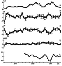Figure 6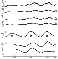Figure 7
Figure 6 shows the initial and smoothed by the L interval annual values of the vector of the interplanetary magnetic field BI and its three orthogonal components Bx, By, and Bz. Comparing variations of the latter with the former makes it possible to conclude that there is no coordination in their occurrence: the vector variations demonstrate the 11-year cyclisity, such cyclisity being absent in the components. At the same time, the BI variations agree well with the modulus values of the vector and the component module (Figure 7). Also the variations of the By and Bx components (particularly the former which occur in phase with the IMF variations and so may be used as an analog of the initial IMF variations near the Earth) are well correlated with the variation of the total magnetic field of the Sun which is shown at the bottom of Figure 6.

Figure 7 shows variations of the modules of the IMF vector and components and also variations of the solar magnetic fields. Here the identity of the variations of the vector and its components with the variations of Bs is already visually seen.Figure 8
Figure 8 shows the results of consideration of the relation between BI and Bs on the correlation plane separately for each cycle. The adjacent points are connected by line, and the arrows and dates show the motion direction in time. The absence of phase shifts between two fields is seen even from this figure. The linear approximation of this distribution results in a high correlation coefficient ( r = 0.950.06 ) and a regression equation BI = (5.20.15) + (6.020.46)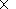10-5 Bs , where BI is in nT and Bs is in Gs (1 Gs  = 105 nT). The following conclusions were derived from the analysis performed:

1. The amplitude attenuation of the cyclic variation of the modulus of the total magnetic field of the Sun near the Earth is a factor of2104.

2. The amplitude of the ~11-year cycle of IMF increases from cycle 20 to cycle 22 and is approximately equal in cycles 21 and 22 (even is slightly higher in the latter), this fact being principally different from the amplitude modulation of the V and n cycles.

3. No phase shifts between the BI and Bs cycles were discovered.

4. The cyclic variation of the modulus of the total magnetic field of the Sun ( Bs ) is the main source of the cyclic variation of IMF.

5. The variations of the initial annual values of BI and its components do not contain explicitly expressed cyclisity with T11-22 years. The best correlation is detected between the phase of the total solar magnetic field variation with the By component of the interplanetary magnetic field.

6. The scatter of points relative the approximating line in Figure 8 has a tendency to be lower in the middle part of the cycle and to increase in the epochs of its extremes.

### 4. Discussion

The cyclic variation of Bs contains a superposition of the |1B| and |2B| magnetic fields; the |1Bs| input prevails in the amplitude variations and the |2Bs| input is manifested in the phase shift of the Bs cycle relative the W cycle by 1-2 years [Rivin, 1998b, 1999a, 1999b]. The corresponding properties of the cyclisity of the solar magnetic fields are "inherited" by the modulus of the interplanetary magnetic field, that is, the variations of BI with T11 years are on the whole identical to similar variations of Bs . At the same time the variations of the By and Bx (the latter in antiphase) components of the initial IMF correspond to the variations of  Bs.

It is principally important and in some way unexpected that the cyclic variations of the two main dynamic parameters of SW, probably first, differ from the variations of BI and, second, have somewhat different properties: the phase of the V cycle is shifted by ~3 year relative the phase of the W cycle, whereas the phase of the n cycle coincides with the phase of the |2Bs| magnetic field cycle. Probably, this difference indicates similar but slightly different formation sources of the cycles of these two parameters. At the same time the amplitude attenuation of the cycles of both parameters shows that probably the field |2Bs| influences each of them more than the |1Bs| field, that is, the plasma has a much deeper origin.

Annual mean values of n before the early 1970s are systematically underestimated by 3-4 cm -3. It follows from the " n-W " plot (Figure 4) that this underestimation is not caused by random errors. It is not yet clear whether it manifests a systematic error in the n determination in King catalogue for the corresponding year or has some physical sense. The results of this paper correct partially the assumptions (made by Rivin  earlier) on the cyclisity nature of the interplanetary medium parameters varying with T11 years.

#### Acknowledgments

This work was performed under partial financing by the Russian Foundation for Basic Research (projects 99-02-18346, 99-05-64296) and the Astronomy program (project 4-264). The authors thank A. E. Levitin for his help.

### References

Obridko, V. N., and Yu. R. Rivin, Magnetic field in the near-equatorial solar photosphere, Astron. Zh. (in Russian), 73, 812, 1996.

Parker, E. N., Basic mechanisms of solar activity, in Proceedings of the Symposium 71 IAU, Prague, August 1975, edited by V. Bumba and I. Klechek, D. Reidel, Norwell, Mass., 1976.

Rivin, Yu. R., Earth's and Sun's Cycles (in Russian), 165 pp., Nauka, Moscow, 1989.

Rivin, Yu. R., Specification of the analytical model of the cyclic variations of the magnetic fields at middle and low latitudes of the Sun, Izv. Akad. Nauk Ross. Ser. Fiz. (in Russian), 63, 1263, 1998a.

Rivin, Yu. R., Principal construction elements of the mechanism of cyclic changes generation in large-scale and local solar magnetic fields, Izv. Akad. Nauk Ross. Ser. Fiz. (in Russian), 63, 1867, 1998b.

Rivin, Yu. R., Principal contradiction between the experimental data on solar activity cyclisity and current theoretical models of its description, Pis'ma Astron. Zh. (in Russian), 25 (4), 314, 1999a.

Rivin, Yu. R., 11-year cycles of the low-latitude large-scale solar magnetic field, its origin and sources in the convection zone, Sol. Phys., 187, 207, 1999b.

Severnyy, A. B., Magnetic fields of the Sun and stars, Usp. Fiz. Nauk (in Russian), 88, 3, 1966.

Load files for printing and local use. This document was generated by TeXWeb (Win32, v.1.3) on February 17, 2003.